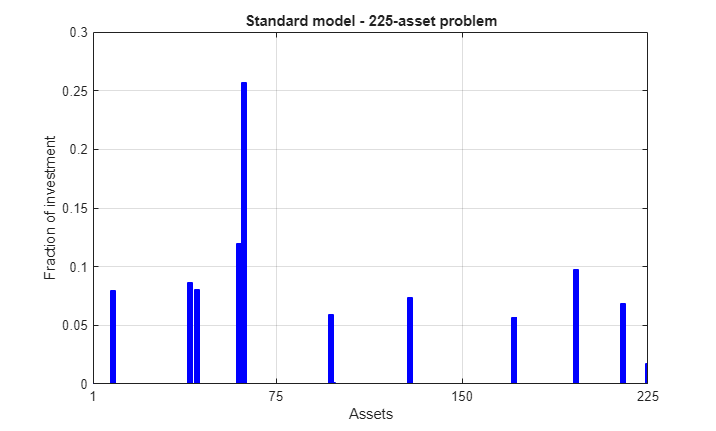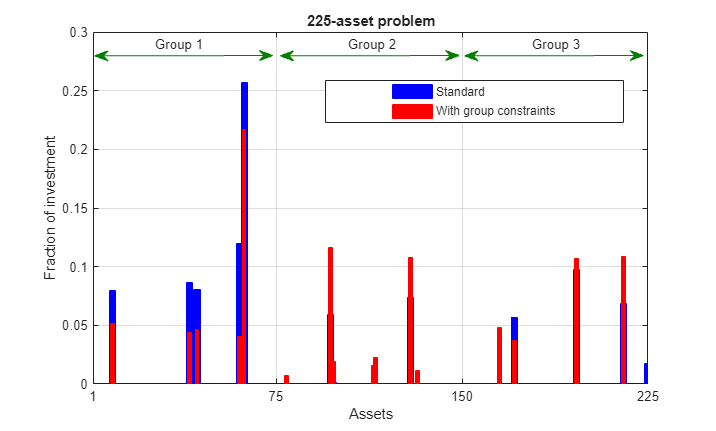# Quadratic Programming for Portfolio Optimization, Problem-Based

This example shows how to solve portfolio optimization problems using the problem-based approach. For the solver-based approach, see Quadratic Programming for Portfolio Optimization Problems, Solver-Based.

Suppose that a portfolio containsdifferent assets. The rate of return of assetis a random variable with expected value. The problem is to find what fractionto invest in each assetin order to minimize risk, subject to a specified minimum expected rate of return.

Letdenote the covariance matrix of rates of asset returns.

The classical mean-variance model consists of minimizing portfolio risk, as measured bysubject to a set of constraints.

The expected return should be no less than a minimal rate of portfolio returnthat the investor desires,the sum of the investment fractions's should add up to a total of one,and, being fractions (or percentages), they should be numbers between zero and one,Since the objective to minimize portfolio risk is quadratic, and the constraints are linear, the resulting optimization problem is a quadratic program, or QP.

### 225-Asset Problem

Let us now solve the QP with 225 assets. The dataset is from the OR-Library [Chang, T.-J., Meade, N., Beasley, J.E. and Sharaiha, Y.M., "Heuristics for cardinality constrained portfolio optimisation" Computers & Operations Research 27 (2000) 1271-1302].

We load the dataset and then set up the constraints for the problem-based approach. In this dataset the rates of returnrange between -0.008489 and 0.003971; we pick a desired returnin between, e.g., 0.002 (0.2 percent).

Load dataset stored in a MAT-file.

load('port5.mat','Correlation','stdDev_return','mean_return') 

Calculate the covariance matrix from correlation matrix.

Covariance = Correlation .* (stdDev_return * stdDev_return'); nAssets = numel(mean_return); r = 0.002; % number of assets and desired return 

### Create Optimization Problem, Objective, and Constraints

Create an optimization problem for minimization.

portprob = optimproblem; 

Create an optimization vector variable 'x' with nAssets elements. This variable represents the fraction of wealth invested in each asset, so should lie between 0 and 1.

x = optimvar('x',nAssets,'LowerBound',0,'UpperBound',1); 

The objective function is 1/2*x'*Covariance*x. Include this objective into the problem.

objective = 1/2*x'*Covariance*x; portprob.Objective = objective; 

The sum of the variables is 1, meaning the entire portfolio is invested. Express this as a constraint and place it in the problem.

sumcons = sum(x) == 1; portprob.Constraints.sumcons = sumcons; 

The average return must be greater than r. Express this as a constraint and place it in the problem.

averagereturn = dot(mean_return,x) >= r; portprob.Constraints.averagereturn = averagereturn; 

### Solve 225-Asset Problem

Set some options, and call the solver.

Set options to turn on iterative display, and set a tighter optimality termination tolerance.

options = optimoptions('quadprog','Display','iter','TolFun',1e-10); 

Call solver and measure wall-clock time.

tic [x1,fval1] = solve(portprob,'Options',options); toc 
 Iter Fval Primal Infeas Dual Infeas Complementarity 0 7.212813e+00 1.227500e+02 1.195948e+00 2.217295e-03 1 8.160874e-04 3.615084e-01 3.522160e-03 2.250524e-05 2 7.220766e-04 3.592574e-01 3.500229e-03 3.378157e-05 3 4.309434e-04 9.991108e-02 9.734292e-04 2.790551e-05 4 4.734300e-04 5.551115e-16 7.771561e-16 4.242216e-06 5 4.719034e-04 6.661338e-16 3.122502e-16 8.002618e-07 6 3.587475e-04 4.440892e-16 3.035766e-18 3.677066e-07 7 3.131814e-04 8.881784e-16 3.686287e-18 9.586695e-08 8 2.760174e-04 7.771561e-16 1.463673e-18 1.521063e-08 9 2.345751e-04 1.110223e-15 1.138412e-18 4.109608e-09 10 2.042487e-04 1.221245e-15 1.084202e-18 6.423267e-09 11 1.961775e-04 1.110223e-16 9.757820e-19 6.068329e-10 12 1.949281e-04 4.440892e-16 9.215718e-19 4.279951e-12 Minimum found that satisfies the constraints. Optimization completed because the objective function is non-decreasing in feasible directions, to within the value of the optimality tolerance, and constraints are satisfied to within the value of the constraint tolerance. Elapsed time is 0.292806 seconds. 

Plot results.

plotPortfDemoStandardModel(x1.x)### 225-Asset Problem with Group Constraints

We now add to the model group constraints that require that 30% of the investor's money has to be invested in assets 1 to 75, 30% in assets 76 to 150, and 30% in assets 151 to 225. Each of these groups of assets could be, for instance, different industries such as technology, automotive, and pharmaceutical. The constraints that capture this new requirement areAdd group constraints to existing equalities.

grp1 = sum(x(1:75)) >= 0.3; grp2 = sum(x(76:150)) >= 0.3; grp3 = sum(x(151:225)) >= 0.3; portprob.Constraints.grp1 = grp1; portprob.Constraints.grp2 = grp2; portprob.Constraints.grp3 = grp3; 

Call solver and measure wall-clock time.

tic [x2,fval2] = solve(portprob,'Options',options); toc 
 Iter Fval Primal Infeas Dual Infeas Complementarity 0 7.212813e+00 1.227500e+02 3.539920e-01 5.253824e-03 1 7.004556e-03 2.901399e+00 8.367185e-03 2.207460e-03 2 9.181962e-04 4.095630e-01 1.181116e-03 3.749424e-04 3 7.515047e-04 3.567918e-01 1.028932e-03 3.486333e-04 4 4.238346e-04 9.005778e-02 2.597127e-04 1.607718e-04 5 3.695008e-04 1.909891e-04 5.507829e-07 1.341881e-05 6 3.691407e-04 6.146337e-07 1.772508e-09 6.817457e-08 7 3.010636e-04 7.691892e-08 2.218223e-10 1.837302e-08 8 2.669065e-04 1.088252e-08 3.138350e-11 5.474712e-09 9 2.195767e-04 8.122574e-10 2.342425e-12 2.814320e-08 10 2.102910e-04 2.839773e-10 8.189470e-13 1.037476e-08 11 2.060985e-04 6.713696e-11 1.936133e-13 2.876950e-09 12 2.015107e-04 0.000000e+00 8.131516e-19 1.522226e-10 13 2.009670e-04 4.440892e-16 8.673617e-19 5.264375e-13 Minimum found that satisfies the constraints. Optimization completed because the objective function is non-decreasing in feasible directions, to within the value of the optimality tolerance, and constraints are satisfied to within the value of the constraint tolerance. Elapsed time is 0.162406 seconds. 

Plot results, superimposed on results from previous problem.

plotPortfDemoGroupModel(x1.x,x2.x);### Summary of Results So Far

We see from the second bar plot that, as a result of the additional group constraints, the portfolio is now more evenly distributed across the three asset groups than the first portfolio. This imposed diversification also resulted in a slight increase in the risk, as measured by the objective function (see column labeled "f(x)" for the last iteration in the iterative display for both runs).

### 1000-Asset Problem Using Random Data

In order to show how the solver behaves on a larger problem, we'll use a 1000-asset randomly generated dataset. We generate a random correlation matrix (symmetric, positive-semidefinite, with ones on the diagonal) using the gallery function in MATLAB®.

Reset random stream for reproducibility.

rng(0,'twister'); nAssets = 1000; % desired number of assets 

### Create Random Data

Generate means of returns between -0.1 and 0.4.

a = -0.1; b = 0.4; mean_return = a + (b-a).*rand(nAssets,1); r = 0.15; % desired return 

Generate standard deviations of returns between 0.08 and 0.6.

a = 0.08; b = 0.6; stdDev_return = a + (b-a).*rand(nAssets,1); 

Load the correlation matrix, which was generated using Correlation = gallery('randcorr',nAssets). (Generating a correlation matrix of this size takes a while, so load the pre-generated one instead.)

load('correlationMatrixDemo.mat','Correlation'); 

Calculate the covariance matrix from correlation matrix.

Covariance = Correlation .* (stdDev_return * stdDev_return'); 

### Create Optimization Problem, Objective, and Constraints

Create an optimization problem for minimization.

portprob2 = optimproblem; 

Create the optimization vector variable 'x' with nAssets elements.

x = optimvar('x',nAssets,'LowerBound',0,'UpperBound',1); 

Include the objective function into the problem.

objective = 1/2*x'*Covariance*x; portprob2.Objective = objective; 

Include the constraints that the sum of the variables is 1 and the average return is greater than r.

sumcons = sum(x) == 1; portprob2.Constraints.sumcons = sumcons; averagereturn = dot(mean_return,x) >= r; portprob2.Constraints.averagereturn = averagereturn; 

### Solve 1000-Asset Problem

Call solver and measure wall-clock time.

tic x3 = solve(portprob2,'Options',options); toc 
 Iter Fval Primal Infeas Dual Infeas Complementarity 0 2.142849e+01 5.490000e+02 3.031839e+00 5.210929e-03 1 9.378552e-03 6.439102e+00 3.555978e-02 6.331676e-04 2 1.128129e-04 3.705915e-03 2.046582e-05 1.802721e-05 3 1.118804e-04 1.852958e-06 1.023291e-08 1.170562e-07 4 8.490176e-05 7.650016e-08 4.224702e-10 7.048637e-09 5 3.364597e-05 4.440892e-16 3.062871e-18 1.037370e-09 6 1.980189e-05 2.220446e-16 8.876905e-19 8.465558e-11 Minimum found that satisfies the constraints. Optimization completed because the objective function is non-decreasing in feasible directions, to within the value of the optimality tolerance, and constraints are satisfied to within the value of the constraint tolerance. Elapsed time is 0.913357 seconds. 

### Summary

This example illustrates how to use problem-based approach on a portfolio optimization problem, and shows the algorithm running times on quadratic problems of different sizes.

More elaborate analyses are possible by using features specifically designed for portfolio optimization in Financial Toolbox™.